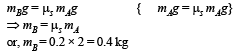Courses

# 28 Year NEET Questions: Laws of Motion- 2

## 30 Questions MCQ Test Physics Class 11 | 28 Year NEET Questions: Laws of Motion- 2

Description
This mock test of 28 Year NEET Questions: Laws of Motion- 2 for NEET helps you for every NEET entrance exam. This contains 30 Multiple Choice Questions for NEET 28 Year NEET Questions: Laws of Motion- 2 (mcq) to study with solutions a complete question bank. The solved questions answers in this 28 Year NEET Questions: Laws of Motion- 2 quiz give you a good mix of easy questions and tough questions. NEET students definitely take this 28 Year NEET Questions: Laws of Motion- 2 exercise for a better result in the exam. You can find other 28 Year NEET Questions: Laws of Motion- 2 extra questions, long questions & short questions for NEET on EduRev as well by searching above.
QUESTION: 1

### Starting from rest, a body slides down a 45° inclined plane in twice the time it takes to slide down the same distance in the absence of friction.The coefficient of friction between the body and the inclined plane is 

Solution:

In presence of friction a = (g sinθ – μg cosθ)

∴   Time taken to slide down the plane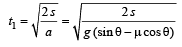In absence of friction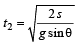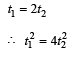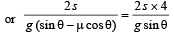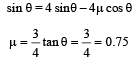QUESTION: 2

### Two bodies of masses m and 4m are moving with equal kinetic energies. The ratio of their linear momenta will be 

Solution: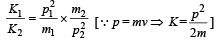Hence,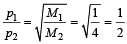QUESTION: 3

### A body of mass 5 kg explodes at rest into three fragments with masses in the ratio 1 : 1 : 3. The fragments with equal masses fly in mutually perpendicular directions with speeds of 21 m/s.The velocity of heaviest fragment in m/s will be

Solution:

Masses of the pieces are 1, 1, 3 kg. Hence

(1 x 21)2 + (1 x 21)2 = (3 x V)2

That is,QUESTION: 4

A 4 kg mass and 1 kg are moving with equal kinetic energies. The ratio of the magnitudes of their linear momenta is 

Solution: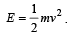. Hence, mv = (2mE)1/2. For same

KE, momentum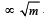Hence, the ratio is 2 : 1.

QUESTION: 5

A 600 kg rocket is set for a vertical firing. If the exhaust speed is 1000 ms–1 , the mass of the gas ejected per second to supply the thrust needed to overcome the weight of rocket is 

Solution:

Thrust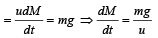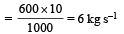QUESTION: 6

A particle of mass m is moving with a uniform velocity v1. It is given an impulse such that its velocity becomes v2. The impulse is equal to 

Solution:

Impulse = final momentum – initial momentum = m (v2 – v1)

QUESTION: 7

A heavy uniform chain lies on horizontal table top. If the coefficient of friction between the chain and the table surface is 0.25, then the maximum fraction of the length of the chain that can hang over one edge of the table is 

Solution:

The force of friction on the chain lying on the table should be equal to the weight of the hanging chain.
Let ρ = mass per unit length of the chain
µ = coefficient of friction
l = length of the total chain
x = length of hanging chain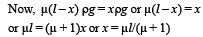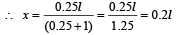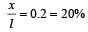QUESTION: 8

Physical independence of force is a consequence of

Solution:

Newton’s first law of motion is related to physical independence of force.

QUESTION: 9

Consider a car moving along a straight horizontal road  with a speed of 72 km/h. If the coefficient of static friction between the tyres and the road is 0.5, the shortest distance in which the car can be stopped is (taking g = 10 m/s2) 

Solution:

Here u = 72 km/h = 20 m/s; v = 0;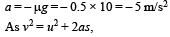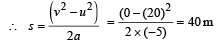QUESTION: 10

A particle of mass M is moving in a horizontal circle of radius R with uniform speed V. When it moves from one point to a diametrically opposite point, its 

Solution:

On the diametrically opposite points, the velocities have same magnitude but opposite directions. Therefore, change in momentum is MV – (– MV) = 2MV

QUESTION: 11

A monkey is decending from the branch of a tree with constant acceleration. If the breaking strength is 75% of the weight of the monkey, the minimum acceleration with which monkey can slide down without breaking the branch is 

Solution:

Let T be the tension in the branch of a tree when monkey is descending with acceleration a. Then mg – T = ma; and T = 75% of weight of monkey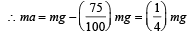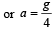QUESTION: 12

A block has been placed on an inclined plane with the slope angle θ, block slides down the plane at constant speed. The coefficient of kinetic friction is equal to 

Solution:

When the block slides down the plane with a constant speed, then the inclination of the plane is equal to angle of repose (θ).
Coeff. of friction = tan of the angle of repose = tanθ.

QUESTION: 13

A shell is fired from a cannon, it explodes in mid air, its total 

Solution:

When shell explodes in mid air its chemical energy is partly converted into mechanical energy, hence K.E. increases.

QUESTION: 14

A satellite in a force free space sweeps stationary interplanetary dust at a rate (dM/dt) = αv. The acceleration of satellite is 

Solution:

Thrust on the satellite,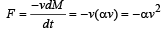Acceleration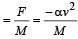QUESTION: 15

If the force on a rocket moving with a velocity of 300 m/sec is 345 N, then the rate of combustion of the fuel, is 

Solution:

Velocity of the rocket (u) = 300 m/s and force (F) = 345N. Rate of combustion of fuel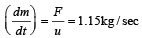QUESTION: 16

What will be the maximum speed of a car on a road turn of radius 30 m if the coefficient of friction between the tyres and the road is 0.4 (Take g = 9.8 m/s2) 

Solution:

r = 30 m and μ = 0.4.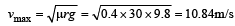QUESTION: 17

A 10 N force is applied on a body produces an acceleration of 1 m/s2. The mass of the body is 

Solution:

By Newton's IInd law of motion,  F = ma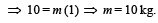QUESTION: 18

A ball of mass 150 g, moving with an acceleration 20 m/s2, is hit by a force, which acts on it for 0.1 sec. The impulsive force is 

Solution:

Mass = 150 gm =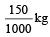Force = Mass × acceleration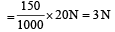Impulsive force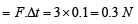QUESTION: 19

A mass of 1 kg is suspended by a thread. It is (i) lifted up with an acceleration 4.9 m/s2, (ii) lowered with an acceleration 4.9 m/s2.The ratio of the tensions is 

Solution:

In case (i) we have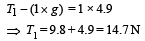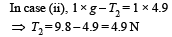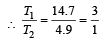QUESTION: 20

A bullet is fired from a gun. The force on the bullet is given by F = 600 – 2 × 105 t where, F is in newton and t in second. The force on the bullet becomes zero as soon as it leaves the barrel.What is the average impulse imparted to the bullet?

Solution:

Given F = 600 – (2 x 105t ) The force is zero at time t, given by
0 = 600 – 2 x 105t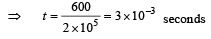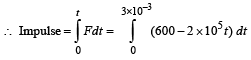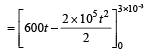= 600 x 3 x 10 –3 – 105 (3 x10 –3)2
= 1.8 – 0.9 = 0.9Ns

QUESTION: 21

A 5000 kg rocket is set for vertical firing. The exhaust speed is 800 ms–1. To give an initial upward acceleration of 20 ms–2, the amount of gas ejected per second to supply the needed thrust will be (g = 10 ms–2) 

Solution:

Given : Mass of rocket (m) = 5000 kg
Exhaust speed (v) = 800 m/s
Acceleration of rocket (a) = 20 m/s2
Gravitational acceleration (g) = 10 m/s2
We know that upward force F = m (g + a) = 5000 (10 +20)
= 5000 × 30 = 150000 N.
We also know that amount of gas ejected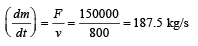QUESTION: 22

Two blocks m1 = 5 gm and m2 = 10 gm are hung vertically over a light frictionless pulley as shown here. What is the acceleration of the masses when they are left free?        (where g is acceleration due to gravity)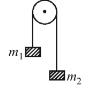Solution: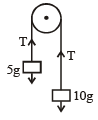Let T be the tension in the string.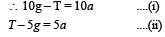Adding (i) and (ii),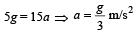QUESTION: 23

A person slides freely down a frictionless inclined plane while his bag falls down vertically from the same height. The final speeds of the man (VM) and the bag (VB) should be such that 

Solution:

As there is only gravitational field which works.
We know it is conservative field and depends only on the end points.  So, VM = VB

QUESTION: 24

A block of mass 1 kg lies on a horizontal surface in a truck the coefficient of static friction between the block and the surface is 0.6. If the acceleration of the truck is 5 m/s2, the frictional force acting on the block is

Solution:

The maximum frictional force acting will be μmg = 6N

But pseudo force acting on the body is ma = 1×5 = 5N

Since pseudo force < frictional force

the frictional force acting is only 5N

QUESTION: 25

If a cricketer catches a ball of mass 150 gm moving with a velocity of 20 m/s, then he experiences a force of (Time taken to complete the catch is 0.1 sec.)

Solution:

Net force experienced =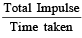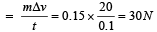QUESTION: 26

A lift weighing 1000 kg is moving upwards with an accelertion of  1 m/s2. The tension in the supporting cable is 

Solution:

T – (1000 × 9.8) = 1000 × 1

⇒  T = 10800N

QUESTION: 27

A 100 N force acts horizontally on a block of 10 kg placed on a horizontal rough surface of coefficient of friction  μ = 0.5. If the acceleration due to gravity (g) is taken as 10 ms–2, the acceleration of the block (in ms–2) is 

Solution: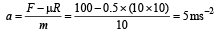QUESTION: 28

A man weighing 80 kg, stands on a weighing scale in a lift which is moving upwards with a uniform acceleration of 5m/s2. What would be the reading on the scale ? (g = 10 m/s2) 

Solution:

Reading of the scale = Apparent wt. of the man = m(g + a) = 80 (10 + 5) = 1200 N

QUESTION: 29

A monkey of mass 20 kg is holding a vertical rope. The rope will not break when a mass of 25 kg is suspended from it but will break if the mass exceeds 25 kg. What is the maximum acceleration with which the monkey can climb up along the rope ? (g = 10 m/s2) 

Solution:

T = Tension caused in string by monkey
= m (g + a)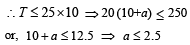QUESTION: 30

The coeffici ent of static friction , μs, between block A of mass 2 kg and the table as shown in the figure is 0.2. What would be the maximum mass value of block B so that the two blocks do not move? The string and the pulley are assumed to be smooth and massless. (g = 10 m/s2)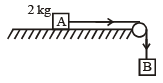Solution: